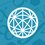# The Truel Saga continues...

I had posted this as a problem but I think my approach to the problem wasn't right.

Three archers A , B and C are standing equidistant from each other, forming an equilateral triangle. Archer A , B and C has 1/3 , 2/3 and 1 probability of hitting the target they aimed for , respectively.

The three archers will play a survival game. The objective of the game for all players is to kill the other two archers and be the only survivor. Deliberate missing is not allowed.

The person who is to shoot a particular shot is to be chosen AT RANDOM among the people alive right before the shot ( which means ABAABBBABAA is possible as is CAAAAA ) .

Assuming that all archers will die if he is hit by an arrow aimed at him, and that all archers will make the best possible optimal moves to maximize their chances of winning (surviving) the probability that Archer A wins can be expressed as a/b where a and b are co-prime positive integers.Note by Santanu Banerjee
6 years, 7 months ago

This discussion board is a place to discuss our Daily Challenges and the math and science related to those challenges. Explanations are more than just a solution — they should explain the steps and thinking strategies that you used to obtain the solution. Comments should further the discussion of math and science.

When posting on Brilliant:

• Use the emojis to react to an explanation, whether you're congratulating a job well done , or just really confused .
• Ask specific questions about the challenge or the steps in somebody's explanation. Well-posed questions can add a lot to the discussion, but posting "I don't understand!" doesn't help anyone.
• Try to contribute something new to the discussion, whether it is an extension, generalization or other idea related to the challenge.

MarkdownAppears as
*italics* or _italics_ italics
**bold** or __bold__ bold
- bulleted- list
• bulleted
• list
1. numbered2. list
1. numbered
2. list
Note: you must add a full line of space before and after lists for them to show up correctly
paragraph 1paragraph 2

paragraph 1

paragraph 2

[example link](https://brilliant.org)example link
> This is a quote
This is a quote
    # I indented these lines
# 4 spaces, and now they show
# up as a code block.

print "hello world"
# I indented these lines
# 4 spaces, and now they show
# up as a code block.

print "hello world"
MathAppears as
Remember to wrap math in $$ ... $$ or $ ... $ to ensure proper formatting.
2 \times 3 $2 \times 3$
2^{34} $2^{34}$
a_{i-1} $a_{i-1}$
\frac{2}{3} $\frac{2}{3}$
\sqrt{2} $\sqrt{2}$
\sum_{i=1}^3 $\sum_{i=1}^3$
\sin \theta $\sin \theta$
\boxed{123} $\boxed{123}$

Sort by:

I got my answer as 297/1120. Hopefully thats not right.

Original Problem 1

- 6 years, 7 months ago

What do you think about following approach?

Strategy for A (when A is chosen):

with probability $p$ he aims at C (tries to kill him),

with probability $1-p$ he deliberately miss his shot.

Calculate the probability that C is killed the first. A and B had been chosen and they always missed C until finally one of them succeeded. Hence the probability that C is killed at step $n+1$ is:

$( \frac {1}{3} \cdot ( p \cdot \frac {2}{3} + ( 1-p ) \cdot \frac {3}{3} ) + \frac {1}{3} \cdot \frac {1}{3} ) ^ n \cdot ( \frac {1}{3} \cdot p \cdot \frac {1}{3} + \frac {1}{3} \cdot \frac {2}{3} ) = ( \frac {4-p} {9} ) ^ n \cdot \frac {p+2}{9}$

Now, after summation of geometric series

$\frac {p+2}{9} \cdot \frac {1}{1 - \frac {4 -p}{9} } = \frac {p+2}{p+5}$

Probability that C is killed the first is $\frac {p+2}{p+5}$ and hence

$1 - \frac {p+2}{p+5} = \frac {3}{ p+5}$

is the probability that B is killed the first.

If C is killed then its duel between A and B. The probability that A wins at step $n+1$ is

$( \frac {1}{2} \cdot \frac {2}{3} + \frac {1}{2} \cdot \frac {1}{3} ) ^ n \cdot ( \frac {1}{2} \cdot \frac {1}{3} ) = ( \frac {1} {2} ) ^ n \cdot \frac {1}{6}$

After summation, the probability that A wins duel with B is

$\frac {1}{6} \cdot \frac {1}{1 - \frac {1}{2} } = \frac {1}{3}$

Finally: A can win in two ways: C is killed and A wins duel with B or B is killed and then A is chosen and A hit C. Hence the probability that A wins is:

$\frac {p+2}{p+5} \cdot \frac {1}{3} + \frac {3}{ p+5} \cdot \frac {1}{2} \cdot \frac{1}{3} = \frac {2p+7}{6p+30}$

The probability that A wins is $\frac {2p+7}{6p+30}$ and it is increasing function on interval $[0,1]$. So, the optimal strategy for A to win is $p=1$ (always aim to opponent ) and maximum probability to win is $\frac {1}{4}$.

- 6 years, 7 months ago